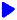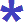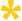Noetic Learning Math Worksheet Creator

Generate Money WorksheetsBack to Worksheet Creator HomeAdding money

Amount is no more than  \$   and is divisible by  cents

Number of questions:  questions per row:   Generate!Subtracting money

Amount is no more than  \$   and is divisible by  cents

Number of questions: questions per row:   Generate!Counting Money    free sample

Include dollar bills  quarters dimes nickels pennies

Number of questions:  questions per row:   Generate!Make Change    free sample

Amount paid is no more than    \$

Number of questions:  questions per row:   Generate!Money multiplication

Money amount is no more than  \$   multiply by  a digits number

Number of questions: questions per row:   Generate!Money division

Money amount is no more than \$   divided by a  digits number

Number of questions:  questions per row:   Generate!